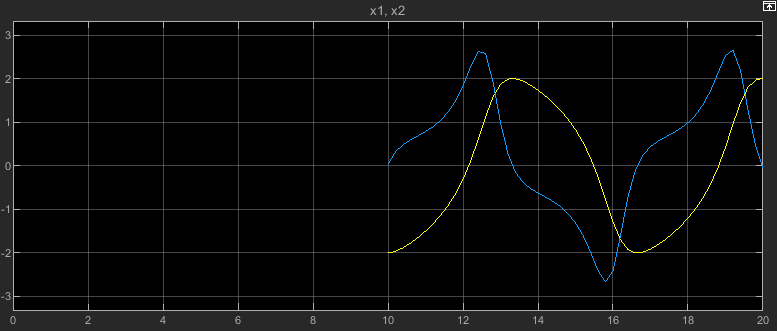# Initial state

Option to specify initial model state or operating point for simulation

Model Configuration Pane: Data Import/Export

## Description

When you load initial states, the simulation starts from the specified state or operating point. You specify the initial state or operating point as a variable or expression. At the start of simulation, the software loads the initial state data.

## Settings

`off` (default) | `on`
`off`

By default, the model does not load initial states or an initial operating point at the start of simulation. The simulation uses default initial states and initial conditions specified in the model.

`on`

The simulation starts from the specified initial state or operating point. In the text box, specify the name of the variable that contains the initial state or operating point. For example, the initial state could be a `Simulink.op.ModelOperatingPoint` object saved from a prior simulation of the same model.

To specify the most complete initial state information, use a `ModelOperatingPoint` object. The model operating point contains complete information about the state of the simulation, including block states, hidden block states, the state of the solver and execution engine, and output values for some blocks. When you use a model operating point as the initial state, the simulation results are the same as a simulation that runs from the beginning. When you specify initial states using logged states data alone, the simulation results might not match. For more information, see Specify Initial State for Simulation.

When you specify logged states data as the initial state for simulation, use the `Dataset`, `Structure`, or ```Structure with time``` format. These formats support several options that the `Array` format does not support:

• Associating initial state values with the path to the block to which the state applies. This association removes dependencies on the block execution order.

• Using different data types for each initial state value.

• Initializing a subset of states.

• Initializing states for a model hierarchy.

The `Array` format is not recommended. When you log states data using the `Array` format, the order of the states within the array depends on the execution order, which can change from one simulation to the next. The `Array` format does not include block path information, so the order of states in the array determines the initial state value associated with each block.

## Examples

expand all

Open the model `vdp`.

```mdl = "vdp"; open_system(mdl);```

Configure the model to save the final operating point at the end of simulation.

1. On the Modeling tab, under Setup, click Model Settings.

2. In the Configuration Parameters dialog box, select the Data Import/Export pane.

3. On the Data Import/Export tab, select Final states and Save final operating point.

4. Click OK.

Alternatively, create a `Simulink.SimulationInput` object to store the parameter values for the simulation. Then, use the `setModelParameter` function to specify the parameter values to use in the simulation.

```simIn = Simulink.SimulationInput(mdl); simIn = setModelParameter(simIn,"SaveFinalState","on"); simIn = setModelParameter(simIn,"SaveOperatingPoint","on");```

Set the stop time for the simulation to 10 seconds. On the Simulation tab, under Simulate, in the Stop Time box, enter `10`, or use the `setModelParameter` function to specify the value of the `StopTime` parameter for the simulation.

`simIn = setModelParameter(simIn,"StopTime","10");`

Simulate the model.

`out = sim(simIn);`

To view the simulation results, double-click the Scope block in the model. The plot in the Scope displays the values of the signals `x1` and `x2` over the 10-second simulation.Resume the simulation by using the operating point you saved at the end of the first simulation as the initial operating point for the second simulation.

Get the final operating point from the first simulation from the `Simulink.SimulationOutput` object `out`.

`vdpOP = out.xFinal;`

Specify the operating point as the initial state for the simulation.

1. On the Modeling tab, under Setup, click Model Settings.

2. In the Configuration Parameters dialog box, on the Data Import/Export pane, select Initial state.

3. In the text box, enter `vdpOP`.

4. Click OK.

Alternatively, create another `Simulink.SimulationInput` object to configure this simulation. Then, use the `setInitialState` function to specify the initial state.

```simIn2 = Simulink.SimulationInput(mdl); simIn2 = setInitialState(simIn2,vdpOP);```

Set the stop time for this simulation to 20. On the Simulation tab, under Simulate, in the Stop Time box, enter `20`, or use the `setModelParameter` function to specify the value of the `StopTime` parameter for the simulation.

`simIn2 = setModelParameter(simIn2,"StopTime","20");`

Simulate the model again, resuming the prior simulation by starting this simulation from the final operating point saved in the first simulation.

`out2 = sim(simIn2);`

The plot in the Scope window updates to show the data from this simulation. The time axis starts at `0` and goes to the simulation stop time of `20`. Because this simulation started from the initial operating point from the first simulation, which ended after 10 simulation seconds, the plot shows the values of the signals `x1` and `x2` only between simulation time 10 seconds and 20 seconds.## Tips

• Initial states loaded using the Initial state parameter override initial conditions and initial values specified in the model.

• To load initial state data for a model that contains bus-valued states, use the `Dataset` format.

• By default, the software issues a warning when you specify initial states using the `Array` format. You can control this diagnostic behavior using the Initial state is array parameter.

• The Initial state parameter does not load initial state data from a data dictionary. When a model uses a data dictionary and you disable model access to the base workspace, the Initial State parameter still has access to resolve variables in the base workspace.

## Recommended Settings

The table summarizes recommended values for this parameter based on considerations related to code generation.

ApplicationSetting
DebuggingNo impact
TraceabilityNo impact
EfficiencyNo recommendation
Safety precautionNo recommendation

## Programmatic Use

 Parameter: `LoadInitialState` Value: `'on'` | `'off'` Default: `'off'` Parameter: `InitialState` Type: string | character vector Value: `Simulink.op.ModelOperatingPoint` object | `Simulink.SimulationData.Dataset` object | structure | matrix Default: `'xInitial'`

## Version History

Introduced before R2006a

expand all